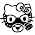## Tuesday, July 26, 2016

### 23 33 52 82 106 | The death of Michael Jordan's father, July 23, 1993 (Jordan breaks silence on social issues, July 23, 2016)

Notice the 9:30 post time.  This is a propaganda article because Michael Jordan is speaking out for two pro-propagnada entities, the police and the NAACP.

Propaganda = 93

Michael Jordan = 113; Mainstream = 113; Dishonest = 113

https://en.wikipedia.org/wiki/James_R._Jordan_Sr.

First notice that Michael Jordan's father was 5'6" supposedly.  Hmmm....

James = 1+1+4+5+1 = 12/21
Raymond = 9+1+7+4+6+5+4 = 36
Jordan = 1+6+9+4+1+5 = 26
Sr. = 1+9 = 10/19
James Raymond Jordan = 74/83 (Masonic = 74) (83, the 23rd prime)
James Raymond Jordan Sr. = 84/93/102

James = 10+1+13+5+19 = 48
Raymond = 18+1+25+13+15+14+4 = 90
Jordan = 10+15+18+4+1+14 = 62
Sr = 19+18 = 37
James Raymond Jordan = 200
James Raymond Jordan Sr. = 237 (He would died July 23, or 23/7)

7/31/1936 = 7+31+19+36 = 93 (The year of his death) (Saturn) (Jordan was in '93)
7/31/1936 = 7+31+(1+9+3+6) = 57 (Died just before 57th birthday)
7/31/1936 = 7+3+1+1+9+3+6 = 30 (Saturn)
7/31//36 = 7+31+36 = 74 (James Raymond Jordan)

7/23/1993 = 7+23+19+93 = 142 (Forty-Two)
7/23/1993 = 7+23+(1+9+9+3) = 52 (Prophecy) (Authority) (Government)
7/23/1993 = 7+2+3+1+9+9+3 = 34
7/23/93 = 7+23+93 = 123 (Conspiracy)

Where he died connects to 'prophecy', just like the date.  That was Bennetsville, SC.

Michael Jordan's father would be murdered exactly 33-days after Jordan won the NBA Finals for the third year in a row; '91, '92 and '93.

Notice those finals ended on June 20, written 6/20 or 20/6.

The day Jordan spoke out, July 23, 2016, was 23-years and 2-days after the death of his father.

7/23/2016 = 7+23+20+16 = 66 (Bulls = 66) (Shoes = 66)
7/23/2016 = 7+23+(2+0+1+6) = 39
7/23/2016 = 7+2+3+2+0+1+6 = 21 (Bulls = 12/21/66)
7/23/16 = 7+23+16 = 46 (Chicago = 46)

In the 66th Book of the Bible, Revelation, Michael is the leader of the Angles of Heaven.

Michael = 33; Thirty-Three = 66
He won 3, then he won 3 more

Update:  March 27, 2017

Also, the man who is credited with killing Jordan has interesting name Gematria.

There's 82-games in an NBA season.  Jordan also won the college championship in '82.

2/17/63 = 2+17+63 = 82

1.Mason scumbag killed his father or his father had to fufill his contract.

2.Mason scumbag killed his father or his father had to fufill his contract.

3.5'6" could be either 56 or 66.
7/23/1993 to 7/25/2016 (when this story actually broke)
23 years and 2 days
322

1.This comment has been removed by the author.

2.66 inches is 167.64 cm

3.Satan = 232 (Jewish Gematria)
James R. Jordan = 56

4.5.Thank you for the assist, Sir Z!

4.Prince Harry is saying HE "Wished He Hadn't Remained Silent About His Mother's Death" -- I sense a theme building here ...

Also noticed that Jordan donated \$1 million to the *INTERNATIONAL* Assoc. of Chiefs of Police -- that sure has a cozy UN sound to it ... IACP ... or ... IAOCOP?? :D ;D

1.Yeah, I'm with you. I'm sure we haven't heard the last from The British Royal Family. Also sure they'll be continuing the push for the Global Cops... but baby steps, federal police first yes?
Sad to see the frog in the boiling water is still yet unaware. *sigh*

5.This quote by Michael Jordan "I can no longer stay silent. "
has been circulating heavy.

I can no longer stay silent = 271 (58th prime)

58 = (Freemasonry) (father)

6.I also believe in the coming month's with this Jordan issue we will see similiar stuff as we did in 97 with the Mar 27, 1997 · Mass suicide involved sedatives, vodka and ... and black Nike athletic shoes ... a UFO was hiding behind the Hale-Bopp Comet. Not to mention I do believe if I'm not mistaken JORDAN as stated in the Bible somewhere that plays a key role in the end days.

7.This comment has been removed by the author.

8.Being that these deaths and dates are so precise; I'm a lil confused as to whether it means, the person is ACTUALLY DEAD, or is it a FAKE death and stash....Like TUPAC! HAHA...Seriously, so hard to tell the fuckery is perpetrated so damn well!